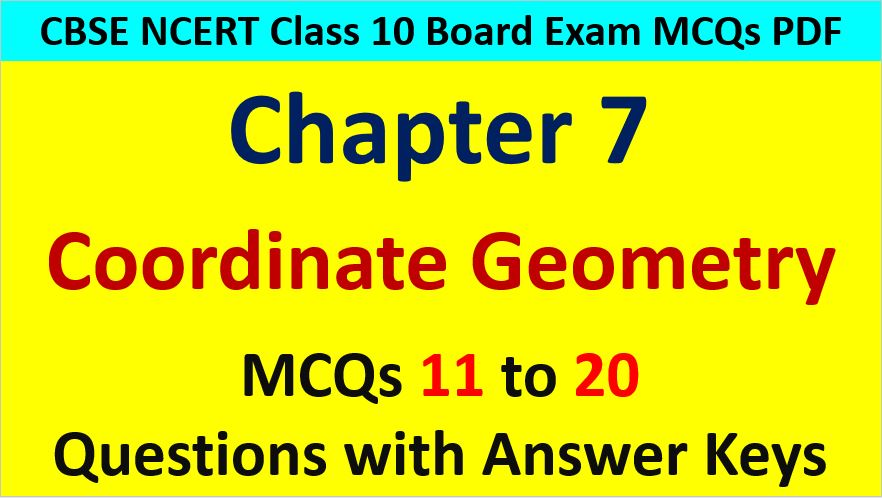Friday, October 22, 2021
Home > CBSE Class 10 > Coordinate Geometry Class 10 Maths MCQ Questions with Answer Keys

# Coordinate Geometry Class 10 Maths MCQ Questions with Answer KeysCoordinate Geometry MCQ Questions for Class 10 Question No 11:

If the line segment joining the points (a, b) and (c, d) subtends at right angle at the origin, then

Option A : ac – bd = 0

Option B : ac + bd = 0

Option C : ab + cd = 0

Option D : ab – cd = 0

Option B : ac + bd = 0

Coordinate Geometry MCQ Questions for Class 10 Question No 12:

AOBC is a rectangle whose three vertices are A(0, 3), O(0, 0) and B(5, 0). Then, the length of its diagonal is

Option A : 5

Option B : 3

Option C : √34

Option D : 4

Option C : √34

Coordinate Geometry MCQ Questions for Class 10 Question No 13:

The perimeter of a triangle with vertices (0, 4), (0, 0) and (3, 0) is

Option A : 5

Option B : 12

Option C : 11

Option D : 7 + √5

Option B : 12

Coordinate Geometry MCQ Questions for Class 10 Question No 14:

If the distance between the points (4, p) and (1, 0) is 5 units, then the value of p is

Option A : 4 only

Option B : ±4

Option C : -4 only

Option D : 0

Option B : ±4

Coordinate Geometry MCQ Questions for Class 10 Question No 15:

If P(1, 2), Q(4, 6), R(5, 7) and S(a, b) are the vertices of a parallelogram PQRS, then

Option A : a = 2, b = 4

Option B : a = 3, b = 4

Option C : a = 2, b = 3

Option D : a = 3, b = 5

Option C : a = 2, b = 3

Coordinate Geometry MCQ Questions for Class 10 Question No 16:

The coordinate of the point which is equidistant from three vertices of a triangle AOB where A(0, 2y), O(0, 0) and B(2x, 0) is

Option A : (x, y)

Option B : (y, x)

Option C : (x/2, y/2)

Option D : (y/2, x/2)

Option A : (x, y)

Coordinate Geometry MCQ Questions for Class 10 Question No 17:

The point P which divides the line segment joining the points A(2, -5) and B(5, 2) in the ratio 2 : 3 lies in the quadrant.

Option A : I

Option B : II

Option C : III

Option D : IV

Option D : IV

Coordinate Geometry MCQ Questions for Class 10 Question No 18:

The vertices of a triangle are (0, 0), (3, 0 and (0, 4). The centroid of the triangle is

Option A : (1/2, 2)

Option B : (1, 4/3)

Option C : (1, 1)

Option D : (0, 3)

Option B : (1, 4/3)

Coordinate Geometry MCQ Questions for Class 10 Question No 19:

The points (k+1, 1), (2k+1, 3) and (2k+2, 2k) are collinear if

Option A : k = -1, 2

Option B : k = 1/2, 2

Option C : k = 2, 1

Option D : k = -1/2, 2

Option D : k = -1/2, 2

Coordinate Geometry MCQ Questions for Class 10 Question No 20:

The area (in square units) of the triangle forms by the points A(a, 0), O(0, 0) and B(0, b) is

Option A : ab

Option B : ab/2

Option C : a2b2/2

Option D : b2/2